## Exercises

1. Show that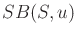cannot be expressed as the union of all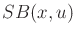for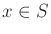.

2. Show that for any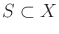and any state transition equation,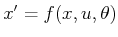, it follows that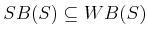.

3. Generalize the strong and weak backprojections of Section 10.1.2 to work for multiple stages.

4. Assume that nondeterministic uncertainty is used, and there is no limit on the number of stages. Determine an expression for the forward projection at any stage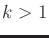, given that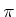is applied.

5. Give an algorithm for computing nondeterministic forward projections that uses matrices with binary entries. What is the asymptotic running time and space for your algorithm?

6. Develop a variant of the algorithm in Figure 10.6 that is based on possibly achieving the goal, as opposed to guaranteeing that it is achieved.

7. Develop a forward version of value iteration for nondeterministic uncertainty, by paralleling the derivation in Section 10.2.1.

8. Do the same as in Exercise 7, but for probabilistic uncertainty.

9. Give an algorithm that computes probabilistic forward projections directly from the plan-based state transition graph,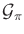.

10. Augment the nondeterministic value-iteration method of Section 10.2.1 to detect and handle states from which the goal is possibly reachable but not guaranteed reachable.

11. Derive a generalization of (10.39) for the case of stage-dependent state-transition equations,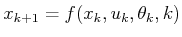, and cost terms,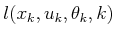, under nondeterministic uncertainty.

12. Do the same as in Exercise 11, but for probabilistic uncertainty.

13. Extend the policy-iteration method of Figure 10.4 to work for the more general case of nature-dependent cost terms,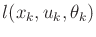.

14. Derive a policy-iteration method that is the nondeterministic analog to the method in Figure 10.4. Assume that the cost terms do not depend on nature.

15. Can policy iteration be applied to solve problems under Formulation 2.3, which involve no uncertainties? Explain what happens in this case.

16. Show that the probabilistic infinite-horizon problem under the discounted-cost model is equivalent in terms of cost-to-go to a particular stochastic shortest-path problem (under Formulation 10.1). [Hint: See page 378 of .]

17. Derive a value-iteration method for the infinite-horizon problem with the discounted-cost model and nondeterministic uncertainty. This method should compute the cost-to-go given in (10.71).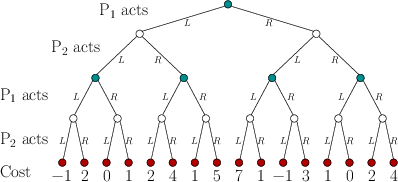18. Figure 10.23 shows a two-stage, zero-sum game expressed as a game tree. Compute the randomized value of this sequential game and give the corresponding randomized security plans for each player.

19. Generalize alpha-beta pruning beyond game trees so that it works for sequential games defined on a state space, starting from a fixed initial state.

20. Derive (10.110) and (10.111).

21. Extend Formulation 2.4 to allow nondeterministic uncertainty. This can be accomplished by specifying sets of possible effects of operators.

22. Extend Formulation 2.4 to allow probabilistic uncertainty. For this case, assign probabilities to the possible operator effects.

Implementations

23. Implement probabilistic backward value iteration and study the convergence issue depicted in Figure 10.3. How does this affect performance in problems for which there are many cycles in the state transition graph? How does performance depend on particular costs and transition probabilities?
24. Implement the nondeterministic version of Dijkstra's algorithm and test it on a few examples.
25. Implement and test the probabilistic version of Dijkstra's algorithm. Make sure that the condition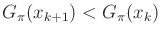from 10.2.3 is satisfied. Study the performance of the algorithm on problems for which the condition is almost violated.
26. Experiment with the simulation-based version of value iteration, which is given by (10.101). For some simple examples, characterize how the performance depends on the choice of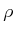.
27. Implement a recursive algorithm that uses dynamic programming to determine the upper and lower values for a sequential game expressed using a game tree under the stage-by-stage model.

Steven M LaValle 2020-08-14